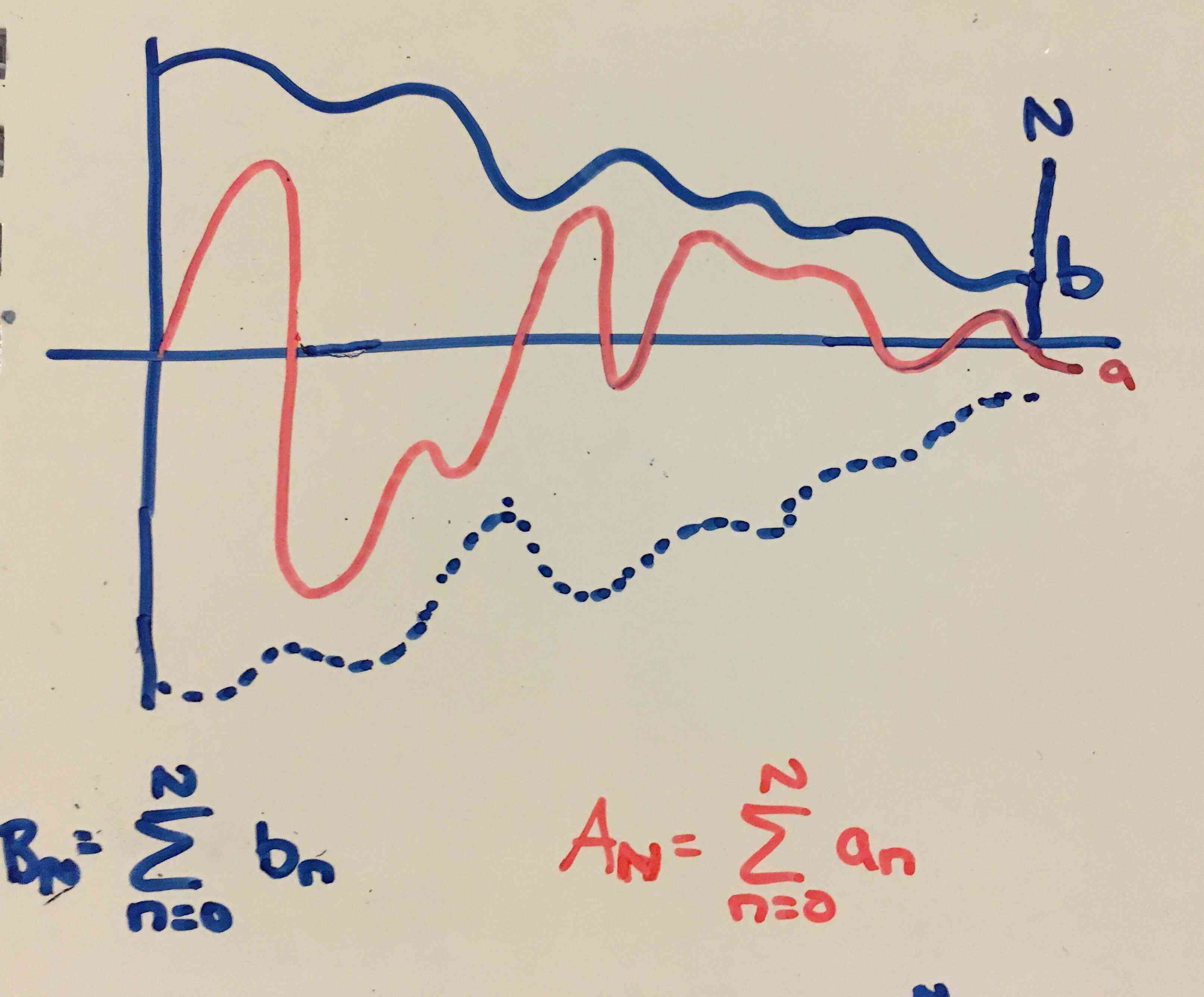Show Question
Math and science::Analysis::Tao::07. Series

# The comparison test (and bounded series of non-negative numbers)

We wish to extend the comparison test for finite series to apply to infinite series.

For finite series, the comparison test appeared as a simple opening lemma (7.1.4 f):

$\text{When } a_i \le b_i \text{ we have } \sum_{i=m}^{n} a_i \le \sum_{i=m}^{n} b_i$

For infinite series, we can only make this statement when $$|a_i| \le b_n$$, otherwise the sum for $$a$$ could diverge. We set this up and prove it below.

First, a useful (and very simple) proposition.

#### Sums of non-negative numbers are bounded iff they are convergent.

Let $$\sum_{n=m}^{\infty}a_n$$ be a formal series of non-negative real numbers. Then this series is convergent if and only if there is a real number $$M$$ such that

$\sum_{n=m}^{N}a_n \le M \text{ for all integers } N \ge m$

#### Proof

Two earlier propositions are:

• Every convergent sequence of real numbers is bounded (6.1.17).
• An increasing sequence which has an upper bound is convergent (6.3.8).

The sequence $$(S_N)_{n=m}^{\infty}$$ representing the series $$\sum_{n=m}^{\infty}a_n$$ is increasing, by definition. So the above two propositions form each side of the proposition's iff statement.

Now we can introduce the comparison test.

### Comparison test

Let $$\sum_{n=m}^{\infty}a_n$$ and $$\sum_{n=m}^{\infty}b_n$$ be two formal series of real numbers, and suppose that $$|a_n| \le b_n$$ for all $$n \ge m$$ (thus all numbers in $$(b_n)$$ must be non-negative). Then if $$\sum_{n=m}^{\infty}b_n$$ is convergent, then $$\sum_{n=m}^{\infty}a_n$$ is absolutely convergent, and in fact

$\left| \sum_{n=m}^{\infty}a_n \right| \le \sum_{n=m}^{\infty}|a_n| \le \sum_{n=m}^{\infty}b_n$

#### Proof

Let $$SA_N := \sum_{n=m}^{N}|a_n|$$ be the Nth partial sum of $$\sum_{n=m}^{\infty}|a_n|$$ and $$SB_N := \sum_{n=m}^{N}b_n$$ be the Nth partial sum of $$\sum_{n=m}^{\infty}b_n$$. As $$\sum_{n=m}^{\infty}b_n$$ is bounded according to the proposition above, and by the the comparison test for finite series we have: $SA_N \le SB_N \le M \text{ for all } N \ge m \text{ and for some bounding real } M$ Through this transitivity, $$(SA_N)_{N=n}^{\infty}$$ also meets the conditions for being bounded. Then from the above propositive again, it must be that $$\sum_{n=m}^{\infty}|a_n|$$ is convergent. The Absolute Convergence Test informs us that absolute convergence implies conditional convergence, and in addition that $$\left| \sum_{n=m}^{\infty}a_n \right| \le \sum_{n=m}^{\infty}|a_n|$$. Thus, with all of the below infinite series converging we can compare them like so:
$\left| \sum_{n=m}^{\infty}a_n \right| \le \sum_{n=m}^{\infty}|a_n| \le \sum_{n=m}^{\infty}b_n$

We can also run the Comparison Test in the contrapositive:

If $$\sum_{n=m}^{\infty}a_n$$ is not absolutely convergent then $$\sum_{n=m}^{\infty}b_n$$ is not conditionally convergent.p171